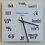# What are the chances?

If you choose the answer to this question at random, what is the probability that it will be correct?

a) $25$ %

b) $50$ %

c) $0$ %

d) $25$ %

Good Luck. :)Note by Muzaffar Ahmed
6 years, 7 months ago

This discussion board is a place to discuss our Daily Challenges and the math and science related to those challenges. Explanations are more than just a solution — they should explain the steps and thinking strategies that you used to obtain the solution. Comments should further the discussion of math and science.

When posting on Brilliant:

• Use the emojis to react to an explanation, whether you're congratulating a job well done , or just really confused .
• Ask specific questions about the challenge or the steps in somebody's explanation. Well-posed questions can add a lot to the discussion, but posting "I don't understand!" doesn't help anyone.
• Try to contribute something new to the discussion, whether it is an extension, generalization or other idea related to the challenge.

MarkdownAppears as
*italics* or _italics_ italics
**bold** or __bold__ bold
- bulleted- list
• bulleted
• list
1. numbered2. list
1. numbered
2. list
Note: you must add a full line of space before and after lists for them to show up correctly
paragraph 1paragraph 2

paragraph 1

paragraph 2

[example link](https://brilliant.org)example link
> This is a quote
This is a quote
    # I indented these lines
# 4 spaces, and now they show
# up as a code block.

print "hello world"
# I indented these lines
# 4 spaces, and now they show
# up as a code block.

print "hello world"
MathAppears as
Remember to wrap math in $$ ... $$ or $ ... $ to ensure proper formatting.
2 \times 3 $2 \times 3$
2^{34} $2^{34}$
a_{i-1} $a_{i-1}$
\frac{2}{3} $\frac{2}{3}$
\sqrt{2} $\sqrt{2}$
\sum_{i=1}^3 $\sum_{i=1}^3$
\sin \theta $\sin \theta$
\boxed{123} $\boxed{123}$

Sort by:

25%

- 6 years, 7 months ago

Why 25% ?

- 6 years, 7 months ago

50%

- 6 years, 7 months ago

Why 50% ?

- 6 years, 7 months ago

25 % . Because there are four options each contributing 25 %

- 6 years, 7 months ago

Not so simple, man, not so simple

- 6 years, 7 months ago

- 6 years, 7 months ago

I guess these sort of questions are good for discussions rather for finding answers. For any answer there is a contradictory statement ......:) how about considering both 25% into single unit and the remaining 0 and 50 we get one more combination 1/3*100

- 6 years, 7 months ago

They are not one unit.. They are different options and the question is about choosing one option randomly...

- 6 years, 7 months ago

25%

- 6 years, 2 months ago

0% is the correct answer .............. if it would have been 25% then if we chose randomly, there are 2 choices out of a total of four , that seem optimal ( i.e. option(a and (d ) so that would mean that the probability would be equal to 50 % ........ Contradictory! if it would have been 50% , then it would have meant that only one choice out of the four is correct i.e. 25%... this answer is also contradictory to itself.......... if it would have been 0% then again it would imply that one choice out of the four is correct i.e. 25% ...... hence it seems that none of the option is correct ......... that leads us to the answer 0% , and hence this answer is not in contrary to itself!!!!!!!

- 6 years, 7 months ago

If 0% is correct, then it is one of the four options, which would give you 25% probability again.

- 6 years, 7 months ago

Agree. Can't be zero percent.If one of the options is correct and the user selects it randomly it definitely will have a probability. I will still stick to 25% . because among 4 options only one is correct and the probability for that becomes 25%(1/4 multiloed by 100)

- 6 years, 7 months ago

There are 2 instances of the option 25%, so if 25% is correct, you will have $\frac{2}{4} \times 100 = 50$ % probability.

- 6 years, 7 months ago

Oop missed it didn't notice 25 repeating again

- 6 years, 7 months ago

50%

- 6 years, 7 months ago